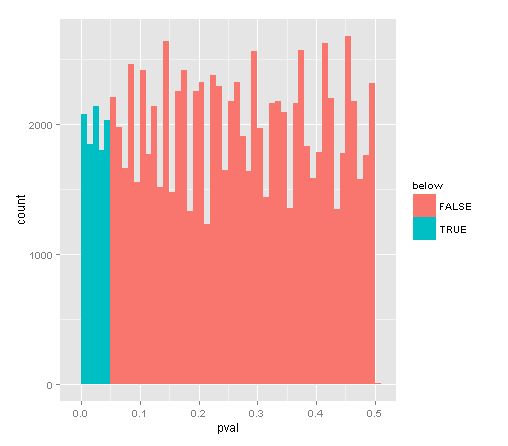#### PROVING THE VALIDITY OF THE Z TEST OF PROPORTIONS WITH MONTE CARLO:

DATA:

           Medication
Symptoms    Drug A Drug B Totals
Heartburn     64     92    156
Normal       114     98    212
Totals       178    190    368

TEST STATISTIC FUNCTION IN R:

For reference: $$Z = \frac{\frac{x_1}{n_1}-\frac{x_2}{n_2}}{\sqrt{p\,(1-p)(1/n_1+1/n_2)}}$$ with $$p = \frac{x_1\,+\,x_2}{n_1\,+\,n_2}$$

z.prop = function(x1,x2,n1,n2){
numerator = (x1/n1) - (x2/n2)
p.common = (x1+x2) / (n1+n2)
denominator = sqrt(p.common * (1-p.common) * (1/n1 + 1/n2))
z.prop.ris = numerator / denominator
return(z.prop.ris)
}

POPULATION SET UP WITH ONE SINGLE (AVERAGE) TRUE PROPORTION:

set.seed(5)

# Number of samples taken to obtain different proportions IF we set it up so that the proportions are actually equal:

samples <- 100000

# Number of cases for Drug A and Drug B:

n1 <- 178
n2 <- 190

# Proportion of patients with heartburn:
p1 <- 64/n1
p2 <- 92/n2

# We will make up a population where the proportion of heartburn suffers is in between p1 and p2:

(c(p <- mean(c(p1, p2)), p1, p2))
 0.4218805 0.3595506 0.4842105

# And we'll take samples of the size of the groups assigned to Drug A and Drug B respectively:

x1 <- rbinom(samples, n1, p)
x2 <- rbinom(samples, n2, p)

# Double - checking that the proportion is kept although the number of successes is different:

(c(mean(x1),mean(x2)))
 75.08542 80.15841
(c(prop1 <- mean(x1)/n1, prop2 <- mean(x2)/n2, p))
 0.4218282 0.4218864 0.4218805

SIMULATION:
# Now we run the z-test in what we just did, but we repeat it 100000 times and put the results in vector pval:

pval <- 0
for (i in 1:100000) {
pval[i] <- pnorm(-abs(z.prop(mean(x1[i]), mean(x2[i]), n1, n2)))
}

mean(pval <= .05)
 0.09887

So what is wrong with this simulation? The value should be below $$0.05$$ and it is not…

The probability integral function dictates that if $$X \sim N(\mu,\sigma^2)$$, the random variable $$Y = \Phi(X)$$ with $$\Phi$$ corresponding to the error function ($$erf$$) (who came up with this name?) will be uniformely distributed as $$Y \sim U(a,b)$$.

Since we know that the $$p-values$$ range from $$(0,1)$$, plotting the histogram should illustrate our expectation (overabundant $$p<0.05$$ values colored in greenish hue):So we are getting just half of the span we expected: from $$[0,0.5]$$, as opposed to $$[0,1]$$. And the problem is in the code treatment of the $$z$$ values produced by the function z.prop. Since $$z$$ is a symmetric distribution the part of the code intended to “look up the probability in the table at the end of the book”: (pnorm(-abs(z.prop(mean(x1[i]), mean(x2[i]), n1, n2)))) is effectively sending all the $$z$$ values above $$0$$ to the negative part of the bell curve, and we end up with double amount of pval < 0.05.

If you happened to get a $$z=1.4$$, “you are calculating $$Pr(Z<-1.4)$$ when you should be calculating $$Pr(Z<-1.4)+Pr(Z>1.4)$$.

This can be fixed by simply doubling the value of the pvalues. Or perhaps more neatly by changing the SIMULATION part of the code in the OP to:

pval <- 0
for (i in 1:100000) {
pval[i] <- pnorm(z.prop(mean(x1[i]), mean(x2[i]), n1, n2))
}
> mean(pval <= .025) + mean(pval >= 0.975)
 0.04923